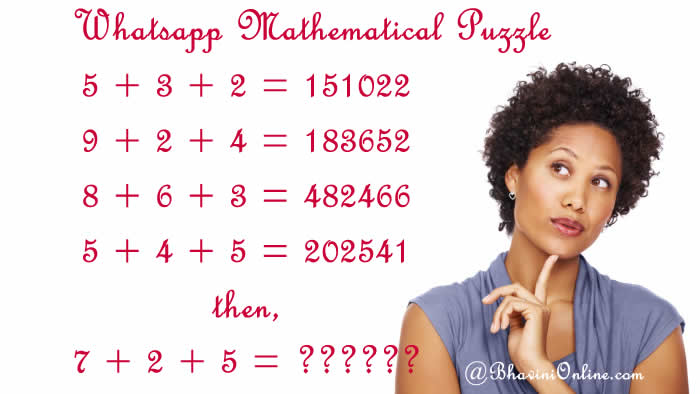# Whatsapp Mathematical Puzzle: If 5+3+2=151022, 7+2+5=?

I came across this tough maths puzzle on Whatsapp today.

Only 1% Students solved it (IAS exam)

5 + 3 + 2 = 151022
9 + 2 + 4 = 183652
8 + 6 + 3 = 482466
5 + 4 + 5 = 202541
then,
7 + 2 + 5 = ??????So were you able to solve the riddle? Leave your answers in the comment section below.

## 3 Replies to “Whatsapp Mathematical Puzzle: If 5+3+2=151022, 7+2+5=?”

1. 143547

14+35
49
49-2
47

thankyou

2. SS gill says:

To find 7 + 2 + 5 = ??????

We use the same formula;
(axb) = (7×2) = 14;
(axc) = (7*5) = 35;
(a*b)+(a*c)-b = (7×2)+(7×5)-2 = 14 + 35 – 2 = 46

Hence 7 + 2 + 5 = 143546